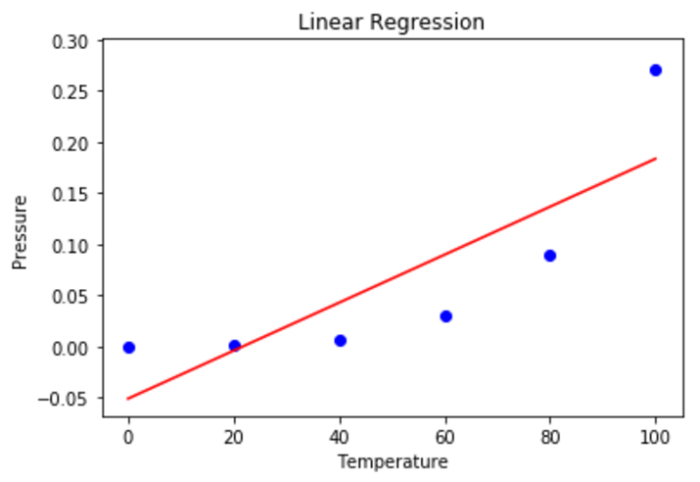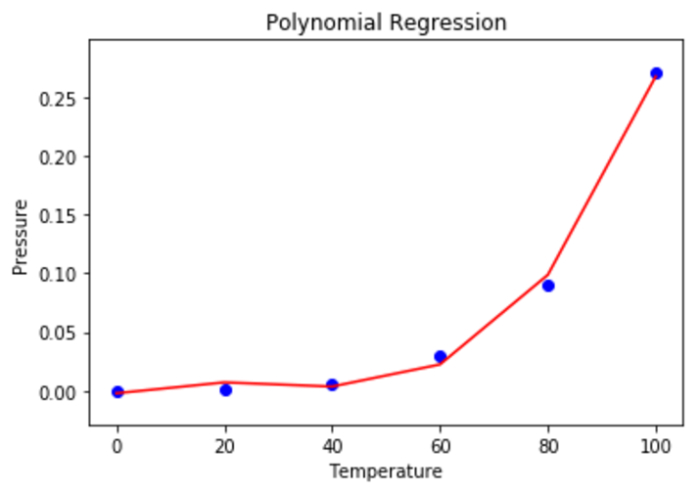# Python Polynomial Regression in Machine Learning

## Introduction

The link between the dependent and independent variables, Y and X, is modelled as the nth degree of the polynomial in polynomial regression, a type of linear regression. In order to draw the best line using data points, this is done. Let’s explore more about the Polynomial regression in this article.

## Polynomial Regression

One of the rare instances of multiple linear regression models is polynomial regression. In other words, it is a sort of linear regression when the dependent and independent variables have a curvilinear connection to one another. In the data, a polynomial connection is fitted.

Additionally, by incorporating several polynomial parts, a number of linear regression equations are transformed into polynomial regression equations.

The relationship between both the independent variable x and the dependent variable y is modelled as an nth degree polynomial in polynomial regression. A nonlinear relationship between both the value of x and the associated conditional mean of y, given by the symbol E(y |x), is fit via polynomial regression.

## Need of Polynomial Regression

A few criteria that specify the requirement for polynomial regression are listed below.

• If a linear model is used to a linear database, as is the case with simple linear regression, a good result is produced. However, a significant output is calculated if this model is applied to a non-linear dataset with no adjustments. These result in increased mistake rates, a drop in accuracy, and an increase inside the loss function.

• Polynomial regression is required in situations when the data points are organized non-linearly.

• A linear model won't cover any data points if a non-linear model is available and we attempt to cover it. In order to guarantee that all of the data points are covered, a polynomial model is employed. Nevertheless, a curve rather than a straight line will work well for most data points when employing polynomial models.

• A scatter diagram of residuals (Y-axis) here on predictor (X-axis) will show regions of many positive residuals inside the middle if we attempt to fit a linear model to curved data. As a result, it is inappropriate in this circumstance.

## Polynomial Regression Applications

Basically, these are employed to define or enumerate non-linear phenomena.

• The rate of tissue growth.

• Progression of pandemic disease.

• Carbon isotope distribution in lake sediments.

Modeling the estimated return of a dependent variable y in relation to the value of an independent variable x is the fundamental aim of regression analysis. We used the equation below in simple regression

y = a + bx + e

Here, the dependent variable is y, along with the independent variables a, b, and e.

## Polynomial Regression Types

Numerous varieties of polynomial regression exist since a polynomial equation's degree has no upper bound and can go up to the nth number. For instance, the second degree of a polynomial equation is typically expressed as a quadratic equation when spoken. As indicated, this degree is valid up to the nth number, and we are free to deduce quite so many equations as we require or want. As a result, polynomial regression is typically categorized as follows.

• When the degree is 1, linear.

• Equation has a quadratic degree of two.

• Depending on the degree used, cubic with a degree as three continues.

When examining the output of chemical synthesis in terms of the temperature where the synthesis takes place, for instance, this linear model will frequently not work out. In such circumstances, we employ a quadratic model.

y = a+b1x+b2+b2+e

Here, the error rate is e, the y-intercept is a, and y is the dependent variable on x.

## Python Implementation of Polynomial Regression

Step 1 − Import datasets and libraries

Import the necessary libraries as well as the dataset for the polynomial regression analysis.

# Importing up the libraries
import numpy as nm
import matplotlib.pyplot as mplt
import pandas as ps
# Importing up the dataset
data


## Output

   sno Temperature Pressure
0    1     0        0.0002
1    2     20       0.0012
2    3     40       0.0060
3    4     60       0.0300
4    5     80       0.0900
5    6     100      0.2700


Step 2 − The dataset is split into two components in step two.

Divide the dataset into the X and y components. X will contain the columns 1 and 2. The two columns will be in column y.

X = data.iloc[:, 1:2].values
y = data.iloc[:, 2].values


Step 3 − Dataset fitting with linear regression

Two components of the linear regression model are fitted.

from sklearn.linear_model import LinearRegressiondata
line2 = LinearRegressiondata()
line2.fit(X, y)


Step 4 − Polynomial Regression Fitting to the Dataset

X and Y are the two components to which the polynomial regression model is fit.

from sklearn.preprocessing import PolynomialFeaturesdata
poly = PolynomialFeaturesdata(degree = 4)
X_polyn = polyn.fit_transform(X)
polyn.fit(X_polyn, y)
line3 = LinearRegressiondata()
line3.fit(X_polyn, y)


Step 5 − In this stage, we are utilizing a scatter plot to visualize the results of the linear regression.

mplt.scatter(X, y, color = 'blue')
mplt.plot(X, lin.predict(X), color = 'red')
mplt.title('Linear Regression')
mplt.xlabel('Temperature')
mplt.ylabel('Pressure')
mplt.show()


## OutputStep 6 − Using a scatter plot to display the polynomial regression findings.

mplt.scatter(X, y, color = 'blue')
mplt.plot(X, lin2.predict(polyn.fit_transform(X)), color = 'red')
mplt.title('Polynomial Regression')
mplt.xlabel('Temperature')
mplt.ylabel('Pressure')
mplt.show()


## OutputStep 7 − Use both linear & polynomial regression to forecast future outcomes. A NumPy 2D array must contain the input variable, it should be noted.

## Linear Regression

predic = 110.0
predicdarray = nm.array([[predic]])
line2.predict(predicdarray)


## Output

Array([0.20657625])


## Polynomial Regression

Predic2 = 110.0
predic2array = nm.array([[predic2]])
line3.predicdict(polyn.fit_transform(predicd2array))


## Output

Array([0.43298445])


• It is capable of doing a wide variety of tasks.

• In general, polynomial suits a large range of curved surfaces.

• The closest representation of the relationship between variables is provided by polynomials.

• These are extremely responsive to deviations.

• The outcomes of a nonlinear analysis might be significantly impacted by the existence of one or two variables.

• Additionally, compared to linear regression, there are unfortunately less model validation techniques available for the discovery of deviations in nonlinear regression.

## Conclusion

We have learned the theory underlying polynomial regression in this article. We learned the implementation of the Polynomial regression.

After applying this model to a real dataset, we could see its graph and utilize it to predict things. We hope this session was beneficial and that we can now confidently apply this knowledge to other datasets.